# Important Miscellaneous Questions for IBPS CLERK & RBI ASSISTANT MAINS – SET 1

Dear banking Aspirant,

The forthcoming examinations are going to be in a different level and you have to get equipped for those examinations. So we are here to provide the necassary things required for your preparations and here we have provided some questions for your miscellaneous preparations.

This section is constantly neglected by many candidates as solving this will take more time and we can solve the other questions to get the quick marks, but in reality some of the easiest questions are from this topic. So prepare for this section and score more in the forthcoming exams.

Q.1) Ratio of present ages of Prian and Naren is 6 : 7. After four years their ages will be in the ratio 7 : 8. 2 years from now,the age of Deepa will be four-fifth of Naren’s age. What is the present age of Deepa?

a) 24 years

b) 28 years

c) 22 years

d) 18 years

e) 20 years

Q.2)If the speed of a boat in still water is 14 km/hr and speed of the stream is half the speed of the boat in still water, find the total time taken to cover  a distance of 147 km upstream and the same distance back.

a) 35 hours

b) 21 hours

c) 28 hours

d) 26 hours

e) None of these

Q.3)  Average marks of Iliyana in five term tests is 88. Average marks of first two term test is 86.5 and the average marks of last two term tests is 89.5. Find her mark in third term test.

a) 78

b) 85

c) 88

d) 86

e) 85.5

Q.4) Three-eigth of a number is equal to 65% of another number. Second number is equal to five-seventh of another number. If the first number is 13, find the third number.

a) 21

b) 10.5

c) 11.5

d) 19

e) 9

Q.5)A man marks the price of a pair of shoes at 12% above the cost price. If he offers a discount of 6%, find his profit percentage?

a) 4.25%

b) 6.2%

c) 5.72%

d) 5.28%

e) 4.96%

Q.6) In a 22 kg mixture, cement and sand are in the ratio 7: 4. If 6 kg of cement is added to this mixture, the ratio of cement and sand in the mixture would be,

a) 5 : 3

b)6 : 5

c)4 : 3

d)3 : 1

e)5 : 2

Q.7) M and P can alone do a piece of work in 8 days and 12 days respectively. With the help of N, they can finish it in 4 days. If the salary difference between M and N is Rs.11440, find the total salary of M, N and P.

a) Rs.22880

b) Rs.34320

c) Rs.17160

d) Rs.28600

e) Rs.40040

Q.8) A sum of Rs.150000 is deposited in a bank at an interest rate of 14% per annum. If the interest is compounded half yearly, find the amount received at the end of 1 year (approximately)

a) Rs.177300

b) Rs.176260

c) Rs.171735

d) Rs.172200

e) Rs.178270

Q.9) If a 330 m long train crosses another train whose length is 60 m less than the first train and travelling in the opposite direction towards each other, in 30 sec, find the sum of the speeds of the two trains.(in km/hr)

a) 72 km/hr

b) 65 km/hr

c) 78 km/hr

d) 75 km/hr

e) 69 km/hr

Q.10)A book seller has 10 painting books, 18 story books and 17 rhymes books. If he sold 2 books, what is the  probability that these two books are both painting books or both story books.

a)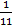b)c)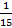d)e) None of these

Check the Answers for the above questions from the one that is given below.

Q.1) c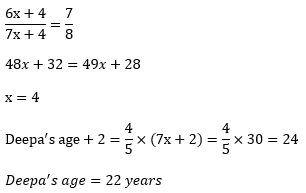Q.2) c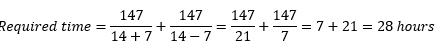Q.3) c

(5×88) = (2×86.5) + x + (2×89.5)

X = 440 – 173 – 179 = 88

Q.4) b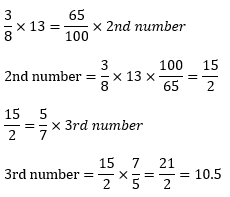Q.5) d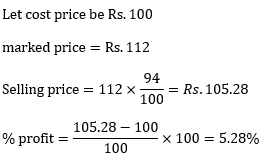Q.6) e

Total mixture (7+4) = 22 kg

1 ratio=2 kg

Original mixture of cement=7(2)=14 kg

Original mixture of sand=4(2)=8 kg

New ratio=(14+6) : 8=20 : 8=5 : 2

Q.7) b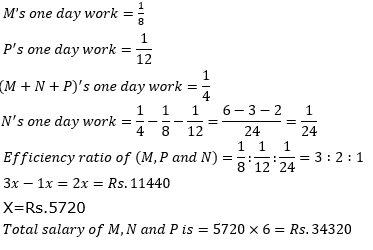Q.8) c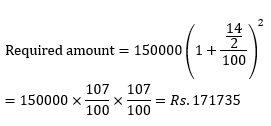Q.9) a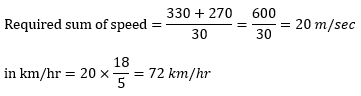Q.10) b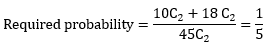Check the Other Important Questions for IBPS CLERK and RBI Assistant Mains from these links.